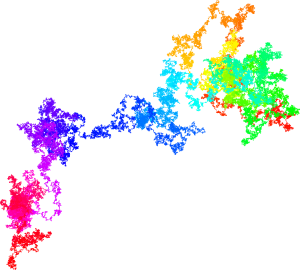# You're reading: Posts Tagged: experimental maths

### 13532385396179 doesn’t climb to a prime

Someone called James Davis has found a counterexample to John H. Conway’s “Climb to a Prime” conjecture, for which Conway was offering \$1,000 for a solution. The conjecture goes like this, as stated in Conway’s list of \$1,000 problems:

Let $n$ be a positive integer. Write the prime factorization in the usual way, e.g. $60 = 2^2 \cdot 3 \cdot 5$, in which the primes are written in increasing order, and exponents of $1$ are omitted. Then bring exponents down to the line and omit all multiplication signs, obtaining a number $f(n)$. Now repeat.

So, for example, $f(60) = f(2^2 \cdot 3 \cdot 5) = 2235$. Next, because $2235 = 3 \cdot 5 \cdot 149$, it maps, under $f$, to $35149$, and since $35149$ is prime, we stop there forever.

The conjecture, in which I seem to be the only believer, is that every number eventually climbs to a prime. The number 20 has not been verified to do so. Observe that $20 \to 225 \to 3252 \to 223271 \to \ldots$, eventually getting to more than one hundred digits without reaching a prime!

Well, James, who says he is “not a mathematician by any stretch”, had a hunch that a counterexample would be of the form $n = x \cdot p = f(x) \cdot 10^y+p$, where $p$ is the largest prime factor of $n$, which in turn motivates looking for $x$ of the form $x=m \cdot 10^y + 1$, and $m=1407$, $y=5$, $p=96179$ “fell out immediately”. It’s not at all obvious to me where that hunch came from, or why it worked.

The number James found was $13\,532\,385\,396\,179 = 13 \cdot 53^2 \cdot 3853 \cdot 96179$, which maps onto itself under Conway’s function $f$ – it’s a fixed point of the function. So, $f$ will never map this composite number onto a prime, disproving the conjecture. Finding such a simple counterexample against such stratospherically poor odds is like deciding to look for Lord Lucan and bumping into him on your doorstep as you leave the house.

A lovely bit of speculative maths spelunking!

via Hans Havermann, whom James originally contacted with his discovery.

### WLTM real number. Must be normal and enjoy long walks on the plane

Something that whipped round Twitter over the weekend is an early version of a paper by Francisco Aragón Artacho, David Bailey, Jonathan Borwein and Peter Borwein, investigating the usefulness of planar walks on the digits of real numbers as a way of measuring their randomness.A million step walk on the concatenation of the base 10 digits of the prime numbers, converted to base 4

A problem with real numbers is to decide whether their digits (in whatever base) are “random” or not. As always, a strict definition of randomness is up to either the individual or the enlightened metaphysicist, but one definition of randomness is normality – every finite string of digits occurs with uniform asymptotic frequency in the decimal (or octal or whatever) representation of the number. Not many results on this subject exist, so people try visual tools to see what randomness looks like, comparing potentially normal numbers like $\pi$ with pseudorandom and non-random numbers. In fact, the (very old) question of whether $\pi$ is normal was one of the main motivators for this study.